# Olympiad Test: Mirror Images And Water Images

## 20 Questions MCQ Test National Cyber Olympiad Class 6 | Olympiad Test: Mirror Images And Water Images

Description
Attempt Olympiad Test: Mirror Images And Water Images | 20 questions in 40 minutes | Mock test for Class 6 preparation | Free important questions MCQ to study National Cyber Olympiad Class 6 for Class 6 Exam | Download free PDF with solutions
QUESTION: 1

Solution:
QUESTION: 2

Solution:
QUESTION: 3

### Choose the correct mirror image of the given figure (X) from amongst the four alternatives.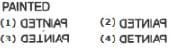Solution:
QUESTION: 4

Choose the correct mirror image of the given figure (X) from amongst the four alternatives.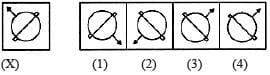Solution:
QUESTION: 5

Choose the correct mirror image of the given figure (X) from amongst the four alternatives.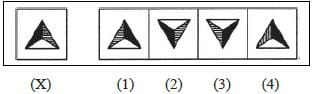Solution:
QUESTION: 6

Choose the correct mirror image of the given figure (X) from amongst the four alternatives.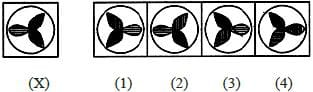Solution:
QUESTION: 7

Choose the correct water image of the given figure (X) from amongst the four alternatives.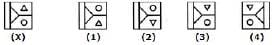Solution:
QUESTION: 8

Choose the correct water image of the given figure (X) from amongst the four alternatives.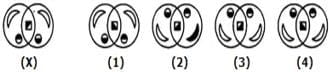Solution:
QUESTION: 9

Choose the correct water image of the given figure (X) from amongst the four alternatives.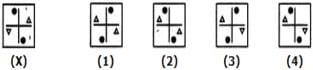Solution:
QUESTION: 10

Choose the alternative which is closely resembles the mirror image of the given combination

BRISK

Solution:
QUESTION: 11

Choose the correct mirror image of the given figure (X) from amongst the four alternatives.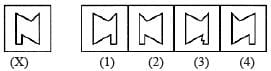Solution:
QUESTION: 12

Choose the correct mirror image of the given figure (X) from amongst the four alternatives.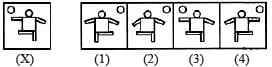Solution:
QUESTION: 13

Choose the correct mirror image of the given figure (X) from amongst the four alternatives.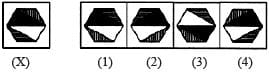Solution:
QUESTION: 14

Choose the correct mirror image of the given figure (X) from amongst the four alternatives.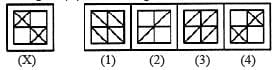Solution:
QUESTION: 15

Choose the correct mirror image of the given figure (X) from amongst the four alternatives.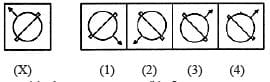Solution:
QUESTION: 16

Choose the correct mirror image of the given figure (X) from amongst the four alternatives.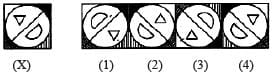Solution:
QUESTION: 17

Choose the correct mirror image of the given figure (X) from amongst the four alternatives.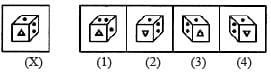Solution:
QUESTION: 18

Choose the correct mirror image of the given figure (X) from amongst the four alternatives.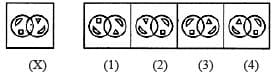Solution:
QUESTION: 19

Choose the correct mirror image of the given figure (X) from amongst the four alternatives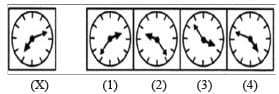Solution:
QUESTION: 20

Choose the correct mirror image of the given figure (X) from amongst the four alternatives.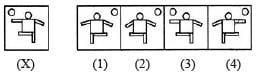Solution:Use Code STAYHOME200 and get INR 200 additional OFF Use Coupon Code